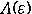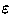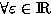### An Algorithmic Approach for the Symmetric Perturbed Eigenvalue Problem Application to the solution of a Schrödinger equation by a kp-Perturbation Method

Claude-Pierre Jeannerod (1), Nicolas Maillard (2), and Eckhard Pflügel (3)

(1) Claude-Pierre Jeannerod LMC-IMAG - (2) Nicolas Maillard LMC-IMAG, Observatoire de Grenoble - (3) Eckhard Pflügel LMC-IMAG

Abstract: In semi-conductor theory, applying the kp-method to the monodimensional Schrödinger equation leads to a symmetric perturbed eigenvalue problem , i.e. to the diagonalization of a matrixdepending on a small parameter, symmetric. The eigenelements ofare expansions in fractional powers of(Puiseux series).

Usually, physicists solve this problem by using Schrödinger perturbation formulas under some restrictive conditions, which make perturbed eigenvector symbolic approximation impossible. This is illustrated by the modified Kane matrix .

To solve this problem completely from a symbolic computing point of view, we consider the symmetric perturbed eigenvalue problem in the case of analytic perturbations [2, 9]. We first review the classical characteristic polynomial approach, showing why it may be not optimal. We also present a direct matricial algorithm : transforming the analytic matrixinto its so-called q-reduced form allows to recover the information we need for the eigenvalues.

This alternative method, as well as the classical one, can be described in terms of the Newton polygon. However, our approach uses only a finite number of terms ofand is more suitable for large matrices and a low approximation order.

Besides, we show that the q-reduction process can simultaneously provide symbolic approximations of both the perturbed eigenvalues and eigenvectors.

The implementation of this algorithm in MAPLE is used to diagonalize the modified Kane matrix up to a given order.

Keywords: perturbation of eigenvalues, symmetric analytic matrices, Newton-Puiseux polygon, q-reduced form of a matrix depending on a parameter, MAPLE package, Schrödinger equation, semi-conductor, kp-method, quantum well.

## References:

1
J. Abdeljaoued. The Berkowitz algorithm, Maple and computing the characteristic polynomial in an arbitrary commutative ring. MapleTech, 4 (3):21-32, 1997.
2
H. Baumgärtel. Analytic perturbation theory for matrices and operators. Birkhauser Verlag, Basel, 1985.
3
C. Cohen-Tannoudji. Mécanique quantique, volumes I et II. Hermann, 1973.
4
D. Duval. Diverses questions relatives au calcul formel avec nombres algébriques. Thèse d'Etat, Faculté des Sciences de Grenoble, 1987.
5
G. Fishman. Cube, Quasi-cube et Würtzite: application au GaN. École thématique du CNRS, Montpellier, 1997.
6
A. Hilali and A. Wazner. Formes super-irréductibles des systèmes différentiels linéaires. Numer. Math., 50:429-449, 1987.
7
C.P. Jeannerod. Algorithmic reduction of matrices depending on a parameter. Technical Report, LMC-IMAG, Grenoble, 1998.
8
E.O. Kane. The kp method. Academic Press, 1967.
9
T. Kato. Perturbation theory for linear operators. Springer, Berlin, 1980.
10
P.O. Löwdin. A note on the quantum-mechanical perturbation theory. Journal of Chemical Physics, 19(11):1396 - 1401, november 1951.
11
M. Monagan. share/linalg/eigen/eigen.mws. system.
12
J. Moro, J.V. Burke, and M.L. Overton. On the Lidskii-Vishik-Lyusternik perturbation theory for eigenvalues of matrices with arbitrary Jordan structure. SIAM J. Matrix Anal. Appl., 18:793-817, 1997.
13
J. Moser. The order of a singularity in Fuchs' theory. Math. Z., pages 379-398, 1960.
14
E. Pflügel. Résolution symbolique des systèmes différentiels linéaires. PhD Thesis, UJF, oct. 1998.
15
M. van Hoeij. The intbasis package. share library.
16
J.H. Wilkinson. The algebraic eigenvalue problem. Oxford, 1965.